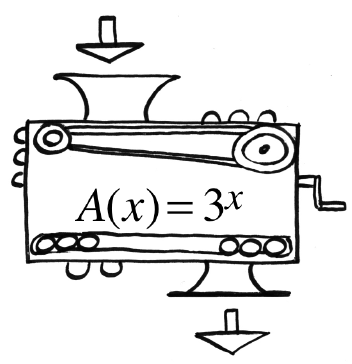### Home > CCA2 > Chapter Ch5 > Lesson 5.1.1 > Problem5-9

5-9.Antonio’s function machine is shown at right. Homework Help ✎

1. What is $A(2)$?

9

2. If 81 came out, what was dropped in?

$3^x=81$

Since $9·9=81$ and $3·3=9$, how many threes will you multiply to make 81?

3. If 8 came out, what was dropped in? Be accurate to two decimal places.

See part (b).

Guess and check the answer.
What is the answer close to?
Later you will learn how to solve an equation like this.